## The Sunday blog: Boost your HMI with advanced mathematics!

### Part 4: Trigonometry

Before you continue reading, I highly recommend that you review the 3 previous episodes of this advanced mathematics blog, because the basics like dealing with fractional numbers on the integer based NEXTION HMIs and drawing simple curves and polynomials are shown and explained there. Today, let’s have a look onto trigonometry: While it remains complicated to approximate arbitrary sine and cosine values on an integer based MCU like the ARM Cortex M0 inside our NEXTION HMI with reasonable precision, methods exist which allow to step from one already known value to the next under the condition that the step is small.

As most of you know, the gradient of a function y = f(x) in a specific point (x0,y0) is given by the value of its derivative y’ = f'(x) in that point. This allows us to approximate a neighbored point located at x0 + d by f(x0 + d) = f(x0) + d * f'(x0). So we can recursively move from one point to the next. This approach is the more precise the more we make the step d small. But smaller d values require naturally more iterations which slows down the curve drawing. You will have to find the best compromise between speed and precision.

Today’s blog project will give you an idea about what can be done with a 3.2″ Standard HMI. As usual, you don’t need to re-create graphics and to copy the example code, the ready-to-use HMI is available for download in this discussion thread of the NEXTION forums.

#### SIN and COS

Knowing that the derivative of sin(x) is cos(x) and the derivative of cos(x) is -sin(x), our recursive calculation is done easily in two lines:
sin = sin + d * cos
cos = cos – d * sin
We only need appropriate initial values to start: sin = 0 and cos = 1. In this HMI project, we use q15 arithmetics to get a maximum of precision, so cos will be initialized to 1<<15 or 32768.

In a first step, let’s trace the sine and cosine function graphs on our HMI. We re-use our drawing canvas of last-week’s blog, but in landscape mode, so that it will be 340px wide. To draw a full period of our functions, we’ll have to map 2 * PI radians onto the 340px. Thus our d value will be 2 * PI / 340 or 606 in the q15 format. I thought that we might have a little more fun adding a slider which would allow us to vary the d value from 606 to 1818, allowing us to draw between 1 and 3 periods of the functions.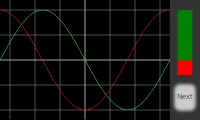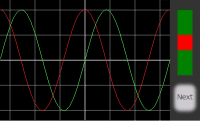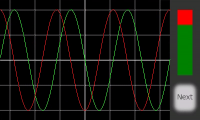First, we define all global variables and constants in the program.s file as follows:

```//The following code is only run once when power on, and is generally used for global variable definition and power on initialization data
int sys0=0,sys1=0,sys2=0 //At present, the definition of global variable only supports 4-byte signed integer (int), and other types of global quantity declaration are not supported. If you want to use string type, you can use variable control in the page to implement
//Trigonometry variables
int sin,cos
//Coordinates, drawing and transformation:
int amp=-100
int x_c=170
int y_c=120
int c_steps=628
int twopi=205783 //This is an approximated value for 2*PI in q15
int round=16384 //This is 0.5 in q15
int c_coeff
int x_max=340
int x,x_last,y,y_last,y_lastsin,y_lastcos,k
page 0 //Power on start page 0```

And now, we add the calculation and drawing code in the slider’s Touch Release event, so that the graphs will be redrawn after each change:

```//Clear screen and set initial condition
ref page0
sin=0
cos=32768 //This is 1 in q15
y_lastsin=sin*amp>>15+y_c
y_lastcos=cos*amp>>15+y_c
for(x=1;x<=x_max;x++)
{
//Calculate new sin and cos
sys0=h0.val*cos>>15
sin+=sys0
sys0=h0.val*sin>>15
cos-=sys0
x_last=x-1
//Draw sine wave
y=sin*amp>>15+y_c
line x_last,y_lastsin,x,y,GREEN
y_lastsin=y
//Draw cosine wave
y=cos*amp>>15+y_c
line x_last,y_lastcos,x,y,RED
y_lastcos=y
doevents // <- Remove this for quicker drawing
}```

And finally, to see already a curve on the screen when opening the page, we add the following Touch release programmatically onto the slider in the Page Post-initialize event:

`click h0,0`

That’s it!

#### Which use?

Drawing sine and cosine curves might not be a primary HMI task unless you want to animate a three-phase motor controller. But we can use the above algorithm to draw for example a circle or an ellipse.
For a circle, the coordinates are:
x(t) = r * sin(t) and y(t) = r * cos(t)
For an ellipse, its only slightly more complex, since we’ll have to use different radii for x and y:
x(t) = rx * sin(t) and y(t) = ry * cos(t)
On the second page of our project, we’ll use the same drawing canvas but we’ll use the slider to vary the x-radius from 50 to 150 while the y radius will be constant at 100px so that everything fits nicely in.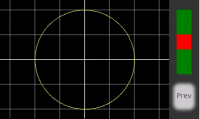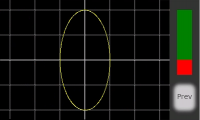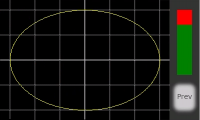The needed constants and variables are already in the program.s file shown above, the drawing trigger in the Page post-initialize event is the same as for the previous page, only the drawing code in the slider’s Touch release event is (naturally) different. While the human eye is more tolerant for minimal pixel errors in curves, it is not tolerant at all when it comes to regular geometric shapes. That’s why this code is a little more complex, adding a rounding helper between the q15 multiplication and the decimal shift:

```//Clear screen and set initial condition
ref page1
sin=0
cos=32768 //This is 1 in q15
c_coeff=twopi/c_steps
x_last=x_c
y_last=-1*cos*amp>>15+y_c
for(k=1;k<=c_steps;k++)
{
//Calculate new sin and cos
sys0=c_coeff*cos+round>>15
sin+=sys0
sys0=c_coeff*sin+round>>15
cos-=sys0
//Draw circle
x=-1*sin*h0.val+round>>15+x_c
y=-1*cos*amp+round>>15+y_c
line x_last,y_last,x,y,YELLOW
x_last=x
y_last=y
doevents // <- Remove this for quicker drawing
}```

You see, as a reader of this blog you can do some miracles on your NEXTION HMI with only about 20 lines of code!

Next week, the geometric shapes based on trigonometry will become still more complex: We’ll meet Mr Jules Antoine Lissajous and his fascinating curves. Thank you for reading – stay tuned!